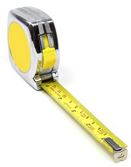# Discrete and Continuous Data

Data can be Descriptive (like "high" or "fast") or Numerical (numbers).

And Numerical Data can be Discrete or Continuous:

Discrete data is counted,
Continuous data is measured

## Discrete Data

Discrete Data can only take certain values.### Example: the number of students in a class

We can't have half a student!### Example: the results of rolling 2 dice

Only has the values 2, 3, 4, 5, 6, 7, 8, 9, 10, 11 and 12

## Continuous DataContinuous Data can take any value (within a range)

Examples:

• A person's height: could be any value (within the range of human heights), not just certain fixed heights,
• Time in a race: you could even measure it to fractions of a second,
• A dog's weight,
• The length of a leaf,
• Lots more!